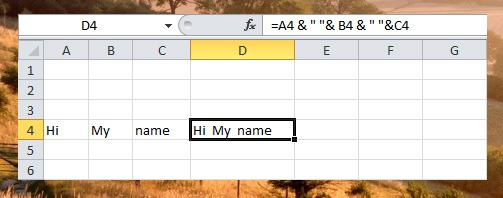# Text Functions

If you want to convert numbers to text or simple text manipulation  in your worksheet, Excel has many text functions that you can use. Like for example, you can easily convert a date in number into a text date format.

Here is the simple syntax of Excel Text functions =TEXT (value, format_text) where value is the number to convert or a cell reference that contain numbers or formula that evaluates to numbers, and format_text is the number format to use. See the example below.You can also use these functions in string manipulation,  Left, Right, Mid, Len, Find, Substitute and Join, let’s take a look at the example one by one.

Left Function

To extract the leftmost characters of the given cell reference with text or string value, use the LEFT() function. In the sample below we extract 3 characters in the string found in Cell A2.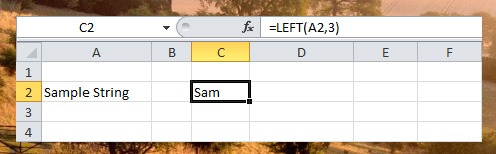Right Function

To extract the rightmost characters of the given cell reference with text or string value, use the RIGHT() function.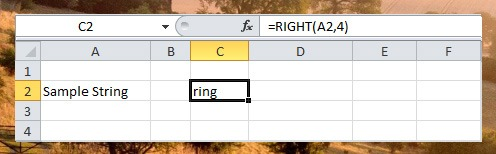Mid Function

To extract the characters in the middle of the given cell reference with text or string value, use the MID() function. In the sample below, we extract 3 characters starting from character position 4.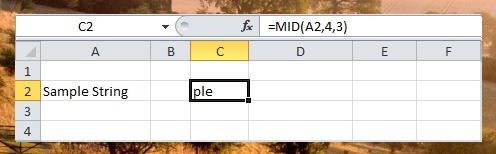Len Function

To get the length of the string in the given cell reference with string value, use LEN() function. Note that space is also counted.Find Function

Use FIND() function to find the position of the substring. In find function the return value is the position of starting character of the given string to find, which in the sample below “S” is found at position 1.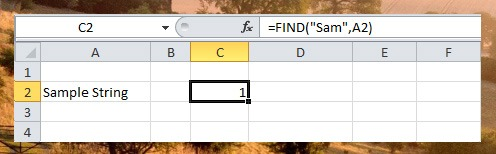Substitute Function

SUBSTITUTE() function is use to replace text in the string.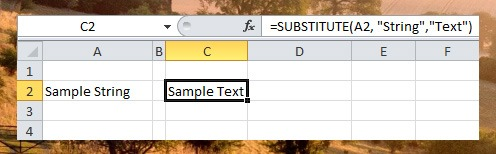Join Strings

If you want to Join the string or text value of two or more cells use the & operator and add ” ” for the space.